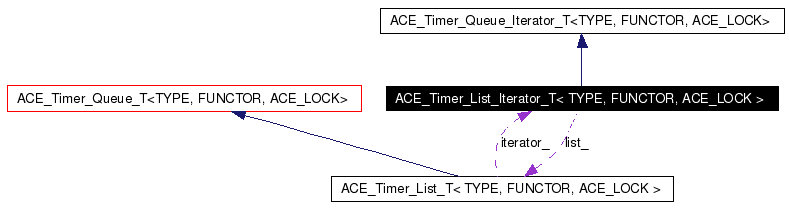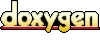# ACE_Timer_List_Iterator_T< TYPE, FUNCTOR, ACE_LOCK > Class Template Reference

Iterates over an ACE_Timer_List. More...

`#include <Timer_List_T.h>`

Inheritance diagram for ACE_Timer_List_Iterator_T< TYPE, FUNCTOR, ACE_LOCK >:[legend]
Collaboration diagram for ACE_Timer_List_Iterator_T< TYPE, FUNCTOR, ACE_LOCK >:[legend]
List of all members.

## Public Types

typedef ACE_Timer_List_T<
TYPE, FUNCTOR, ACE_LOCK >
List

## Public Member Functions

ACE_Timer_List_Iterator_T (List &lst)
Constructor.

virtual ~ACE_Timer_List_Iterator_T (void)
Destructor.

virtual void first (void)
Positions the iterator at the earliest node in the Timer Queue.

virtual void next (void)
Positions the iterator at the next node in the Timer Queue.

virtual int isdone (void) const
Returns true when there are no more nodes in the sequence.

virtual ACE_Timer_Node_T<
TYPE > *
item (void)
Returns the node at the current position in the sequence.

## Protected Attributes

Listlist_
Pointer to the ACE_Timer_List that we are iterating over.

ACE_Timer_Node_T< TYPE > * current_node_
Current position in the ACE_Timer_List.

## Detailed Description

### template<class TYPE, class FUNCTOR, class ACE_LOCK> class ACE_Timer_List_Iterator_T< TYPE, FUNCTOR, ACE_LOCK >

Iterates over an ACE_Timer_List.

This is a generic iterator that can be used to visit every node of a timer queue.

Definition at line 36 of file Timer_List_T.h.

## Member Typedef Documentation

 template typedef ACE_Timer_List_T ACE_Timer_List_Iterator_T< TYPE, FUNCTOR, ACE_LOCK >::List
 Definition at line 40 of file Timer_List_T.h.

## Constructor & Destructor Documentation

 template ACE_Timer_List_Iterator_T< TYPE, FUNCTOR, ACE_LOCK >::ACE_Timer_List_Iterator_T ( List & lst )
 Constructor. Definition at line 19 of file Timer_List_T.cpp. ```00020 : list_ (lst) 00021 { 00022 this->first(); 00023 } ```

 template ACE_Timer_List_Iterator_T< TYPE, FUNCTOR, ACE_LOCK >::~ACE_Timer_List_Iterator_T ( void ) ` [virtual]`
 Destructor. Definition at line 26 of file Timer_List_T.cpp. ```00027 { 00028 } ```

## Member Function Documentation

 template void ACE_Timer_List_Iterator_T< TYPE, FUNCTOR, ACE_LOCK >::first ( void ) ` [virtual]`
 Positions the iterator at the earliest node in the Timer Queue. Definition at line 33 of file Timer_List_T.cpp. ```00034 { 00035 this->current_node_ = this->list_.get_first(); 00036 } ```

 template int ACE_Timer_List_Iterator_T< TYPE, FUNCTOR, ACE_LOCK >::isdone ( void ) const` [virtual]`
 Returns true when there are no more nodes in the sequence. Definition at line 53 of file Timer_List_T.cpp. ```00054 { 00055 return this->current_node_ == 0; 00056 } ```

 template ACE_Timer_Node_T< TYPE > * ACE_Timer_List_Iterator_T< TYPE, FUNCTOR, ACE_LOCK >::item ( void ) ` [virtual]`
 Returns the node at the current position in the sequence. Definition at line 61 of file Timer_List_T.cpp. ```00062 { 00063 if (! this->isdone()) 00064 return this->current_node_; 00065 return 0; 00066 } ```

 template void ACE_Timer_List_Iterator_T< TYPE, FUNCTOR, ACE_LOCK >::next ( void ) ` [virtual]`
 Positions the iterator at the next node in the Timer Queue. Definition at line 41 of file Timer_List_T.cpp. ```00042 { 00043 // Make sure that if we are at the end, we don't wrap around 00044 if (! this->isdone()) 00045 this->current_node_ = this->current_node_->get_next (); 00046 if (this->current_node_ == this->list_.head_) 00047 this->current_node_ = 0; 00048 } ```

## Member Data Documentation

 template ACE_Timer_Node_T* ACE_Timer_List_Iterator_T< TYPE, FUNCTOR, ACE_LOCK >::current_node_` [protected]`
 Current position in the ACE_Timer_List. Definition at line 64 of file Timer_List_T.h.

 template List& ACE_Timer_List_Iterator_T< TYPE, FUNCTOR, ACE_LOCK >::list_` [protected]`
 Pointer to the ACE_Timer_List that we are iterating over. Definition at line 61 of file Timer_List_T.h.

The documentation for this class was generated from the following files:
Generated on Thu Nov 9 11:31:19 2006 for ACE by1.3.6